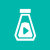IBM 开发的继深蓝之后的新一代大型计算机。 Watson得名于IBM创始人Thomas J. Watson，是当下人工智能的最高端应用。0+0+0+0+0+0+0+0+0+0+0+0+0+0+0+0+0+0+0+1+0+0+0+0+0+0+0+0+0+0+0+0+0+0+0+0+0+0+0+0+0+0+0+0+0+
Top